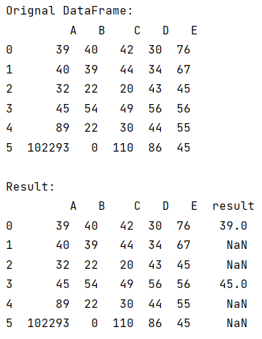# How to Compare Two Columns of Pandas DataFrame?

Given a Pandas DataFrame, we have to compare its two columns. By Pranit Sharma Last updated : September 23, 2023

Pandas is a special tool that allows us to perform complex manipulations of data effectively and efficiently. Inside pandas, we mostly deal with a dataset in the form of DataFrame. DataFrames are 2-dimensional data structures in pandas. DataFrames consists of rows, columns, and the data.

## Problem statement

Given a Pandas DataFrame, we have to compare its two columns.

## Comparing Two Columns of Pandas DataFrame

Comparing columns is important as we need to understand the difference between the columns. For this purpose, we will use a method called numpy.where(), it acts like a conditional statement that checks the condition defined inside it and then compares the columns.

### Syntax

```numpy.where(condition, [x, y, ]/)
```
Note

To work with pandas, we need to import pandas package first, below is the syntax:

```import pandas as pd
```

Let us understand with the help of an example,

## Python Program to Compare Two Columns of Pandas DataFrame

```# Importing pandas
import pandas as pd

# Importing numpy package
import numpy as np

# Create a DataFrame
df = pd.DataFrame({
'A':[39,40,32,45,89,102293],
'B':[40,39,22,54,22,0],
'C':[42,44,20,49,30,110],
'D':[30,34,43,56,44,86],
'E':[76,67,45,56,55,45]
})

# Display original DataFrame
print("Orignal DataFrame:\n",df,"\n")

# Comparing two columns
df['result'] = np.where((df['A'] <= df['B']) & (
df['A'] <= df['C']), df['A'], np.nan)

# Display result
print("Result:\n",df)
```

### Output

The output of the above program is: